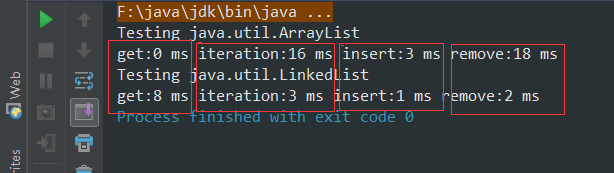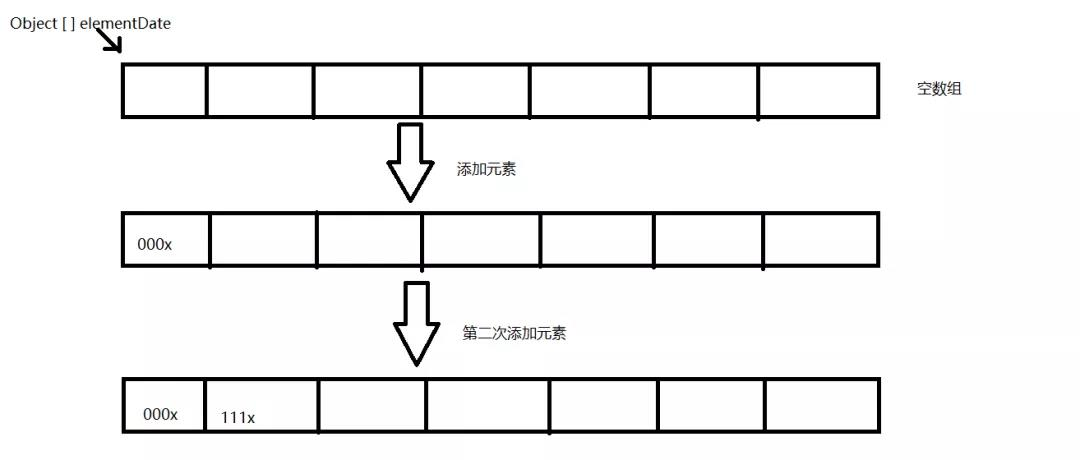+关注继续查看

public class ListPerformance
{
private static final int REPS = 100;

private abstract static class Tester//内部抽象类，作为List测试。
{
String name;
int size;

Tester(String name, int size)
{
this.name = name;
this.size = size;
}

abstract void test(List a);
}

private static Tester[] tests = {new Tester("get", 300)//一个测试数组，存储get(随机取)、iteration(顺序遍历)、insert(中间插入)、remove(随机删除)
{
void test(List a)
{
for (int i = 0; i < REPS; i++) {
for (int j = 0; j < a.size(); j++) {
a.get(j);
}
}
}
}, new Tester("iteration", 300)
{
void test(List a)
{
for (int i = 0; i < REPS; i++) {
Iterator it = a.iterator();
while (it.hasNext()) it.next();
}
}
}, new Tester("insert", 1000)
{
void test(List a)
{
int half = a.size() / 2;
String s = "test";
ListIterator it = a.listIterator(half);
for (int i = 0; i < size * 10; i++) {
}
}
}, new Tester("remove", 5000)
{
void test(List a)
{
ListIterator it = a.listIterator(3);
while (it.hasNext()) {
it.next();
it.remove();
}
}
},
};

public static void test(List a)
{
System.out.println("Testing " + a.getClass().getName());//输出测试的类名称
for (int i = 0; i < tests.length; i++) {
fill(a, tests[i].size);//填充空集合
System.out.print(tests[i].name);
long t1 = System.currentTimeMillis();
tests[i].test(a);//进行测试
long t2 = System.currentTimeMillis();
System.out.print(":" + (t2 - t1)+" ms ");
}
}

public static Collection fill(Collection c, int size)
{
for (int i = 0; i < size; i++) {
}
return c;
}

public static void main(String[] args)
{
test(new ArrayList());
System.out.println();
}

}首先，真的夸一下，这段代码写得真是好啊，无论是内部类的应用还是对面向对象的认识，都考虑的恰到好处，哎，我什么时候才能写出这么棒的代码啊。

测试结果每次都有些许的差异，但不难得出以下的结论：

1、在 ArrayList 中进行随机访问（即 get()）以及循环反复是最划得来的 。原因在于，ArrayList是基于数组而来的，所以每个元素都有其对应的index，所以随机定位一个元素要快捷的多。19 0Java集合性能大比拼，你真的了解经常被使用的它们么？
3993 02812 01286 0919 01418 02492 05736 01903 0
90

0